# 2017-CVPR-PointNet：Deep Learning on Point Sets for 3D Classification and Segmentation

## 2. 引言

PointNet 直接接收点云数据作为输入，输出类别标签（分类）或者每个点的分割标签（分割）。PointNet 结构非常简单，每个点云数据在早期都是进行相同且独立的操作。在基础的设置下，点云的每个点都是表示为 $(x,y,z)$ 坐标；如果想要处理其它特征，则可以再增加其它的维度。

• 设计了一个新的深度神经网络来处理无序的 3D 点云数据；
• 展示了如何训练 PointNet 来处理分类、分割等不同任务；
• 对 PointNet 的稳定性和有效性提供了全面的分析（即理论和实验都有）；
• 对 PointNet 中一些选定的层给出了可视化的图解，并对其性能给出了直观解释。

## 3. 点云上的深度学习

### 3.1 点云属性

• 无序性：因此处理点集的神经网络应当对数据顺序不敏感；
• 点间交互：点云数据由欧拉空间中的坐标点组成，每个点不是各自孤立的，而是相互之间有关联的；
• 变换不变性：对于空间中的几何物体，模型在点云数据上学习到的表示应当具有一定的变换不变性。比如针对点的旋转、平移等变换，学习到的表示应当不发生变化。

### 3.2 模型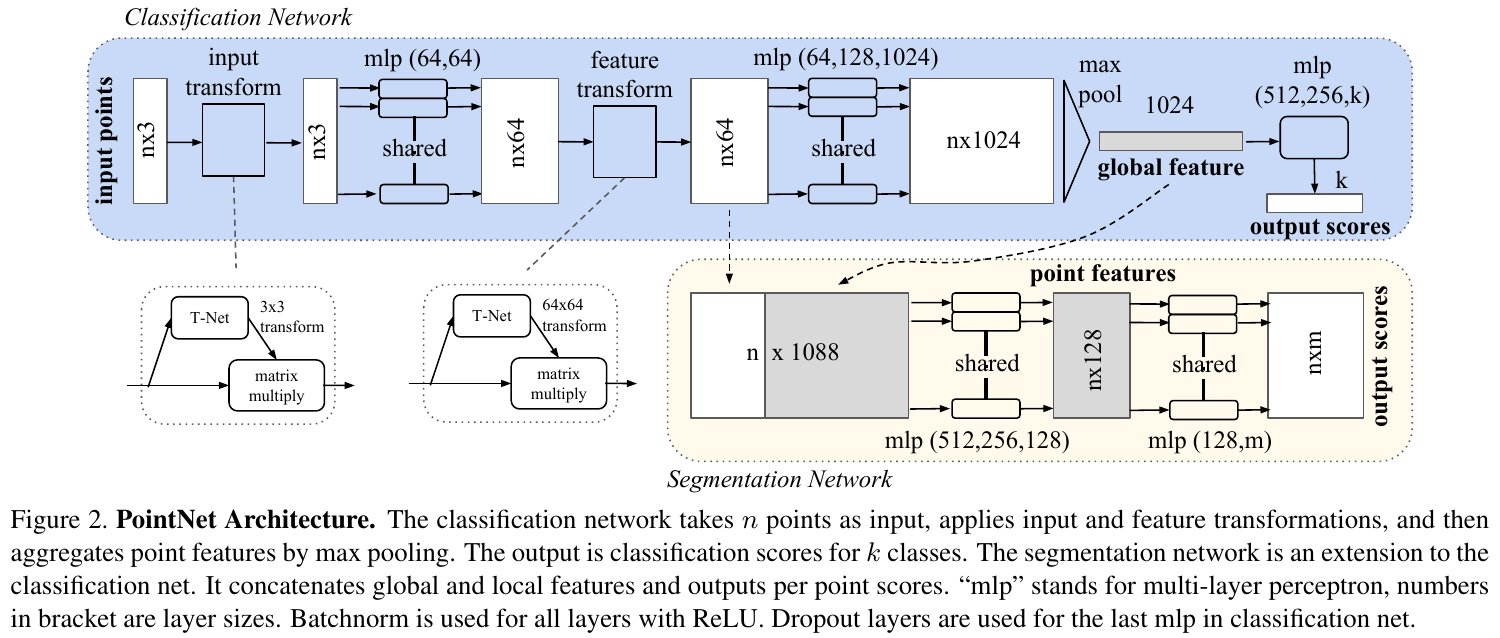PointNet 有三个重要的模块：

• Max Pooling：关于无序数据的对称函数；
• Local and Global Information Combination：聚合局部信息和全局信息；
• Joint Alignment Network：对齐输入点和点特征。

#### 3.2.1 关于无序数据的对称函数

• 按照某种规范对无序数据进行排序；
• 将数据当作有序的序列数据输入 RNN，但考虑数据顺序的所有组合；
• 使用一个简单的对称函数来聚合信息，比如 $+$$*$ 操作就是对称的。

#### 3.2.3 双对齐网络

$L_{r e g}=\left\|I-A A^{T}\right\|_{F}^{2}$

### 3.2.4 理论分析

$\mathcal{X} = \{S: S \subseteq [0, 1]^m, |S| = n\}$$f: \mathcal{X} \rightarrow \mathbb{R}$ 是一个在 $\mathcal{X}$ 的关于 Hausdorff 距离 $d_H(\cdot, \cdot)$ 的连续函数。

$f$ 为关于 $d_H(\cdot,\cdot)$ 的连续函数，即 $\forall \varepsilon > 0, \exist \delta > 0$，对任意的 $S, S^{'} \in \mathcal{X}$，如果 $d_H(S, S^{'}) < \delta$，则有 $|f(S) - f(S^{'}) < \varepsilon$

• 定理一$\forall \varepsilon > 0$，存在一个连线函数 $h$ 和对称函数 $g(x_1, \cdots, x_n) = \gamma \odot \max$，使得对任意的 $S \in \mathcal{X}$，有

$\left\lvert f(S) - \gamma\left(\max_{x_i \in S}\{h(x_i)\}\right)\right\rvert < \varepsilon$

其中，$x_1, \cdots, x_n$$S$ 中的所有元素的一个随意顺序组合，$\gamma$ 是一个连续函数，$\max$ 为向量 element-wise 取最大值操作，其对向量的每一维度取最大值。

这个定理主要说明的是，PointNet 可以拟合任意的点云集合。

• 定理二：假设 $\boldsymbol{u}: \mathcal{X} \rightarrow \mathbb{R}^K$ 使得 $\boldsymbol{u} = \max_{x_i \in S}\{h(x_i)\}$$f = \gamma \odot \boldsymbol{u}$，则有

\begin{align*} &(a)\quad \forall S, \exist \mathcal{C}_S, \mathcal{N}_s \subseteq \mathcal{X}, f(T) = f(S)\quad \text{ if } \mathcal{C}_S \subseteq T \subseteq \mathcal{N}_S \\ &(b)\quad \lvert \mathcal{C}_S \rvert \leq K \end{align*}

这个定理主要说明的是，即使对 PointNet 增加一些输入扰动点，也不会影响其最终的结果。

## 4. 实验

### 4.1 3D 任务

• 3D 物体分类：作者在 ModelNet40 数据集上进行了实验，基本达到了 SOTA 的效果：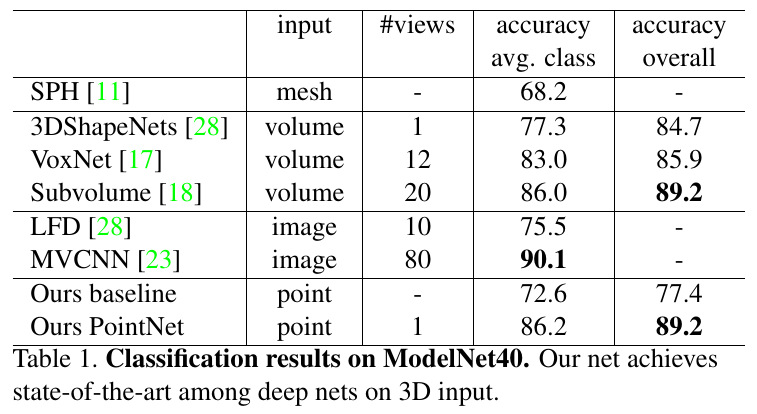• 3D 物体分割： 作者在部分 ShapeNet 数据集上进行了实验，结果如下：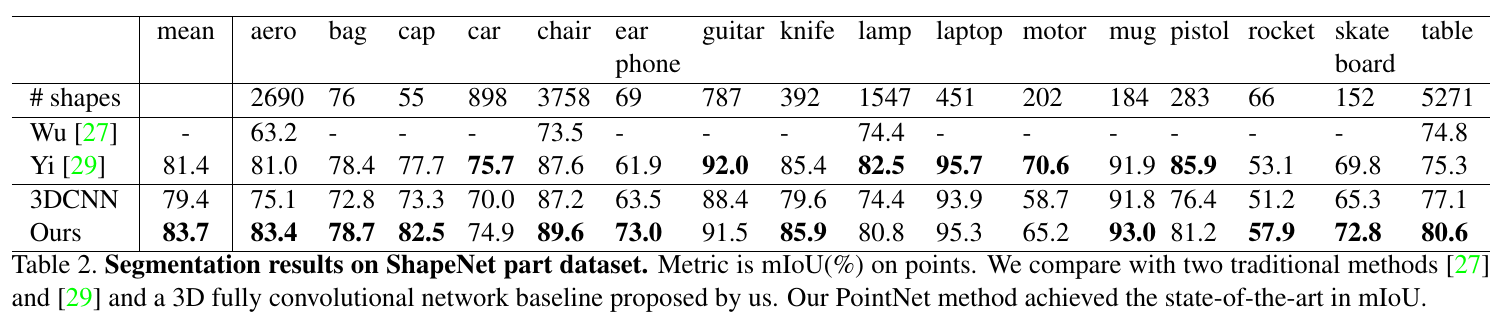• 3D 语义分割：作者在 Stanford 3D semantic 数据集 上进行了实验，结果如下：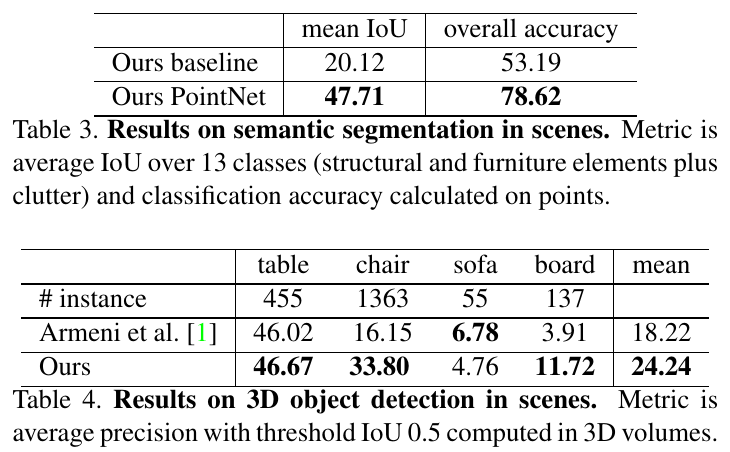其中，baseline 是使用手工设计特征的实验结果。

### 4.2 架构设计分析

• MLP + Max Pooling：作者分别对比了不使用对称函数的三种处理点云数据的方式，即 MLP(unsorted input)、MLP(sorted input) 和 LSTM，以及使用 MLP + 三种不同的对称函数的方式。结果如下图所示，MLP + Max pooling 的方法性能最好：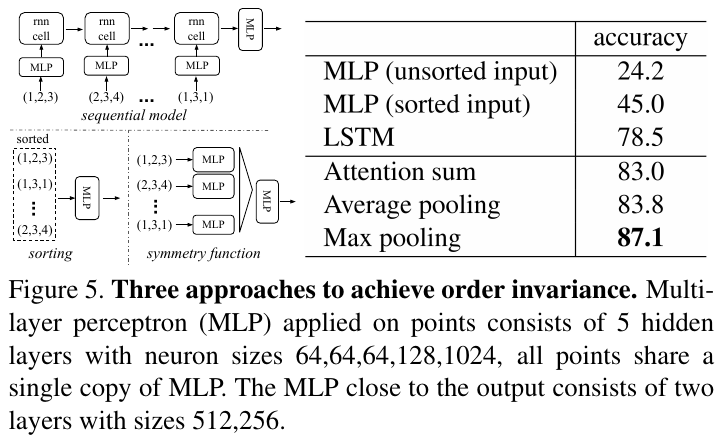• Input Feature Transforms：作者对比了使用 Input Transform 和 Feature Transform，以及对 Feature Transform 加上正则项损失的性能，最后发现综合使用 Input Transform + Feature Transform + Reg Loss 性能最好：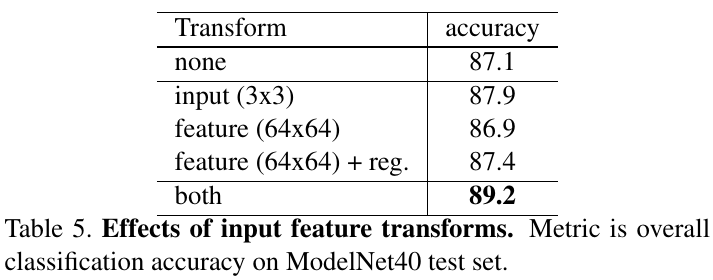• 鲁棒性测试：作者最后 PointNet 网络的鲁棒性能，分别使用了三种方式：丢弃部分点、增加异常点、对点加高斯噪声扰动。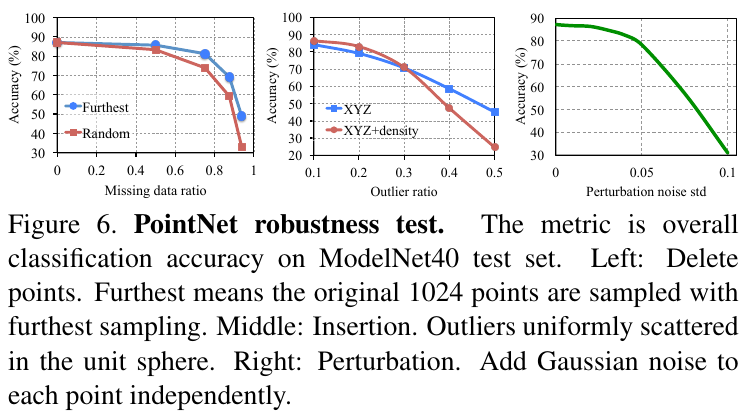### 4.3 可视化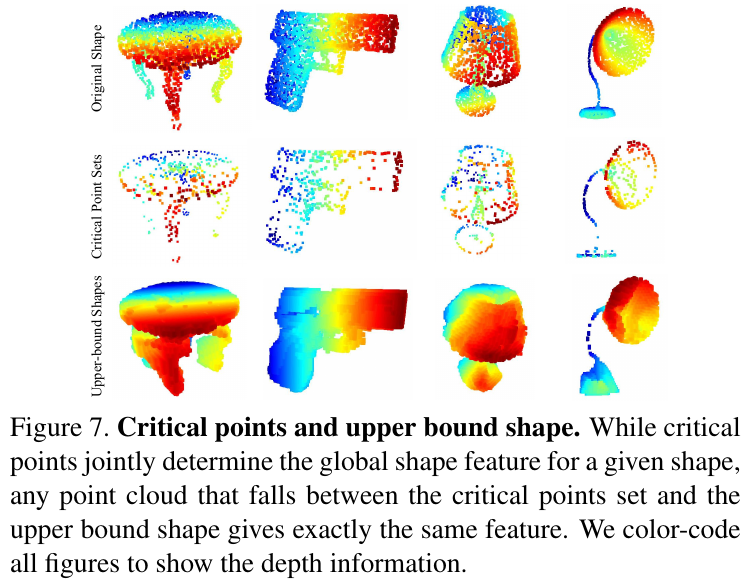### 4.4 复杂度分析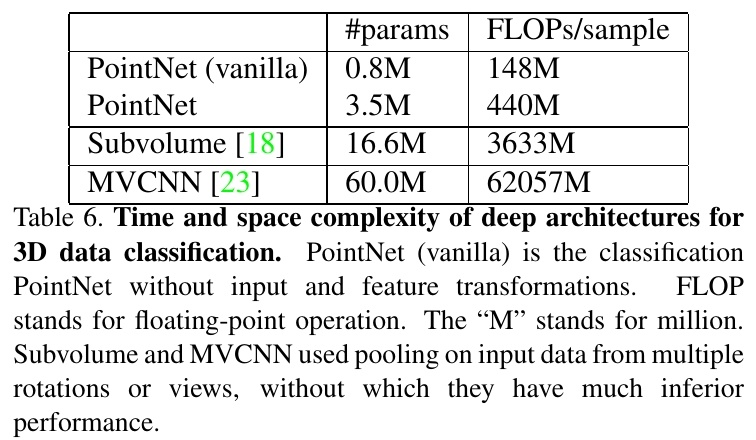## 附录

1. Qi, C. R., Su, H., Mo, K., & Guibas, L. J. (2017). Pointnet: Deep learning on point sets for 3d classification and segmentation. In Proceedings of the IEEE conference on computer vision and pattern recognition (pp. 652-660).
2. Vinyals, O., Bengio, S., & Kudlur, M. (2015). Order matters: Sequence to sequence for sets. arXiv preprint arXiv:1511.06391.